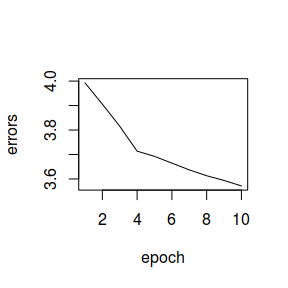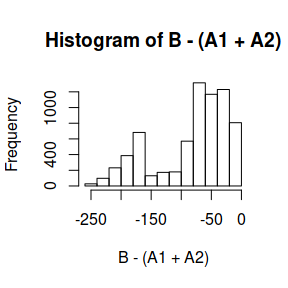# Package

This package includes an example Recurrent Neural Network. The package is loaded using:

library(rnn)
##
## Attaching package: 'rnn'
## The following object is masked _by_ '.GlobalEnv':
##
##     int2bin

# Code

We can view the code of the main rnn() function by calling it without the parathesis (not printed here).

trainr

As can be seen from the above, the model relies on two other functions that are available through the sigmoid package.

The first function is logistic(), which converts an integer to its sigmoid value.

(a <- sigmoid::logistic(3))
##  0.9525741

The code for the sigmoid() function is:

sigmoid::logistic
## function (x, k = 1, x0 = 0)
## 1/(1 + exp(-k * (x - x0)))
## <bytecode: 0x561be787b870>
## <environment: namespace:sigmoid>

The second function converts the sigmoid value of a number to its derivative.

sigmoid::sigmoid_output_to_derivative(a) # a was created above using sigmoid()
##  0.04517666

Finally, we can inspect this code using:

sigmoid::sigmoid_output_to_derivative
## function (x)
## x * (1 - x)
## <bytecode: 0x561be784ef78>
## <environment: namespace:sigmoid>

# Application

An example is included in the help file.

help('trainr')

Below is a basic function that converts integers to binary format (read left to right)

# basic conversion
i2b <- function(integer, length=8)
as.numeric(intToBits(integer))[1:length]

# apply to entire vectors
int2bin <- function(integer, length=8)
t(sapply(integer, i2b, length=length))

First we generate the data:

# create sample inputs
X1 = sample(0:127, 5000, replace=TRUE)
X2 = sample(0:127, 5000, replace=TRUE)

# create sample output
Y <- X1 + X2

# convert to binary
X1 <- int2bin(X1)
X2 <- int2bin(X2)
Y  <- int2bin(Y)

# Create 3d array: dim 1: samples; dim 2: time; dim 3: variables.
X <- array( c(X1,X2), dim=c(dim(X1),2) )
Y <- array( Y, dim=c(dim(Y),1) ) 

This example is:

# train the model
model <- trainr(Y=Y[,dim(Y):1,,drop=F], # we inverse the time dimension
X=X[,dim(X):1,,drop=F], # we inverse the time dimension
learningrate   =  0.1,
hidden_dim     = 10,
batch_size = 100,
numepochs = 10)
## Trained epoch: 1 - Learning rate: 0.1
## Epoch error: 3.99295608836146
## Trained epoch: 2 - Learning rate: 0.1
## Epoch error: 3.90566677897122
## Trained epoch: 3 - Learning rate: 0.1
## Epoch error: 3.81535185392363
## Trained epoch: 4 - Learning rate: 0.1
## Epoch error: 3.71352010816584
## Trained epoch: 5 - Learning rate: 0.1
## Epoch error: 3.69216869206069
## Trained epoch: 6 - Learning rate: 0.1
## Epoch error: 3.66452553610603
## Trained epoch: 7 - Learning rate: 0.1
## Epoch error: 3.63701587773638
## Trained epoch: 8 - Learning rate: 0.1
## Epoch error: 3.61304333777895
## Trained epoch: 9 - Learning rate: 0.1
## Epoch error: 3.59372022922585
## Trained epoch: 10 - Learning rate: 0.1
## Epoch error: 3.57128202445098

See the evolution of the error over different epochs:

plot(colMeans(model\$error),type='l',
xlab='epoch',
ylab='errors'                  )Now create testing data

# create test inputs
A1 = int2bin( sample(0:127, 7000, replace=TRUE) )
A2 = int2bin( sample(0:127, 7000, replace=TRUE) )

# create 3d array: dim 1: samples; dim 2: time; dim 3: variables
A <- array( c(A1,A2), dim=c(dim(A1),2) )

Predict based on testing data.

# predict
B  <- predictr(model,
A[,dim(A):1,,drop=F]
)
B = B[,dim(B):1]

Define basic functions to convert binary to integer

b2i <- function(binary)
packBits(as.raw(c(binary, rep(0, 32-length(binary) ))), 'integer')

bin2int <- function(binary){
binary <- round(binary)
length <- dim(binary)    # determine length of binary representation
apply(binary, 1, b2i)     } # apply to full matrix

Test prediction against true values

# convert back to integers
A1 <- bin2int(A1)
A2 <- bin2int(A2)
B  <- bin2int(B)

# plot the difference
hist( B-(A1+A2) )